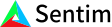1. Bob Anderson
×
IntuitiveML

## Intuition: Perceptrons and Artificial Neural Networks

May 29, 2020

Perceptrons are just neural networks with a single output node, so how a perceptron works and how a neuron in a neural network works are the exact same. For any specific neuron in a neural network, it takes in the weighted sum of all of its inputs and then applies a function to that sum. The function applied to a neuron’s inputs is called an activation function. In the classical formulation for a perceptron, this function is the heaviside function, but for a neural network, this function can be whatever you want.

We can expand upon the perceptron by adding additional layers to it. This is called a multi layer perceptron or an artificial neural network. For every layer in the network, we connect each neuron to every neuron in the layer before it. So for this example, the output node connect to every node in the hidden layer, and every node in the hidden layer connects to every input.

These layers of neurons that connect to every input are called fully connected layers (or FC), and if you combine all of these layers together you get an artificial neural network.